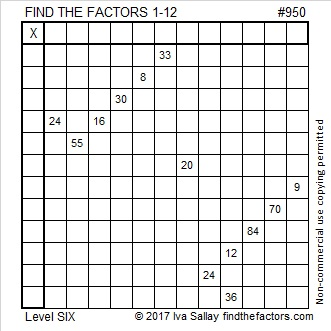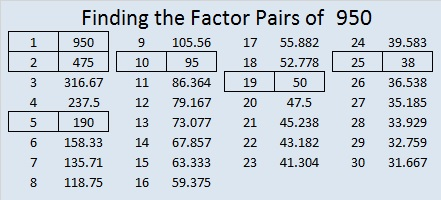# 950 and Level 6

Today’s puzzle is a level 6, so it isn’t for beginners. It is trickier than the easier levels, but don’t let that stop you from giving it a try. If you use guess and check, you will likely become frustrated, but you can solve this puzzle IF you use logic before you write each factor.Print the puzzles or type the solution in this excel file: 12 factors 942-950

950 is the hypotenuse of two Pythagorean triples:
266-912-950 which is (7-24-25) times 38
570-760-950 which is (3-4-5) times 190

950 is 2525 in BASE 7 because 2(7³) + 5(7²) + 2(7¹) + 5(7⁰) = 950

• 950 is a composite number.
• Prime factorization: 950 = 2 × 5 × 5 × 19, which can be written 950 = 2 × 5² × 19
• The exponents in the prime factorization are 2, 1, and 1. Adding one to each and multiplying we get (1 + 1)(2 + 1)(1 + 1) = 2 × 3 × 2 = 12. Therefore 950 has exactly 12 factors.
• Factors of 950: 1, 2, 5, 10, 19, 25, 38, 50, 95, 190, 475, 950
• Factor pairs: 950 = 1 × 950, 2 × 475, 5 × 190, 10 × 95, 19 × 50, or 25 × 38,
• Taking the factor pair with the largest square number factor, we get √950 = (√25)(√38) = 5√38 ≈ 30.82207